# 「Cryptography-Boneh」:Collision Resistance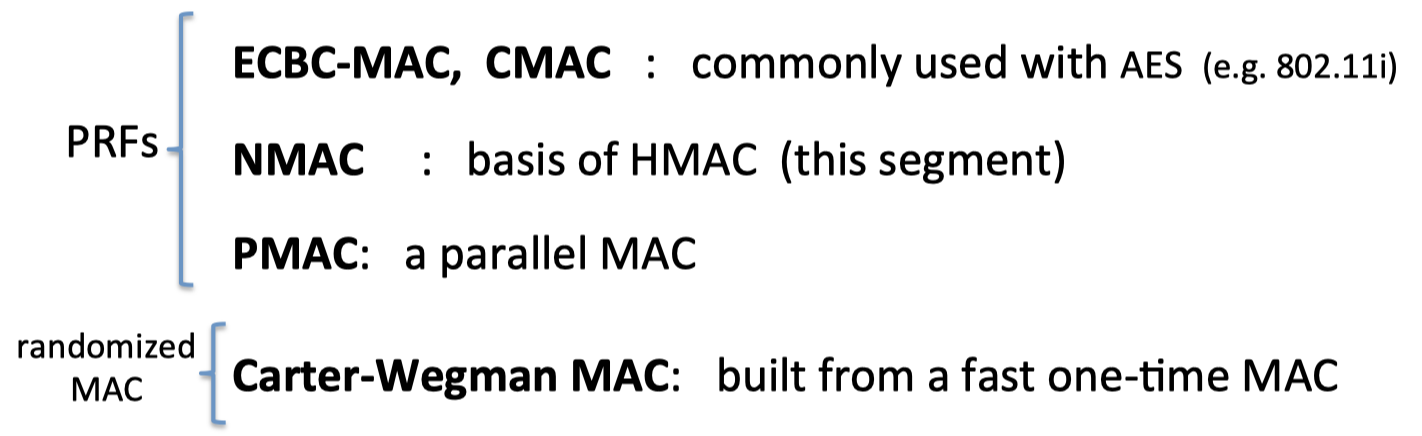# Collision Resistance

Let H: M →T be a hash function (|M|>>|T|)

【H是一个哈希函数】

A collision for H is a pair $\mathrm{m_0,m_1\in M}$ such that:

$$\mathrm{H(m_0)=H(m_1)\quad and\quad m_0\ne m_1}$$

【哈希碰撞是指，存在两个不同的消息，其哈希函数的值相等。】

A function is collision resistant if for all (explicit) “eff” algs. A:

$$\operatorname{Adv}_{\mathrm{CR}}[\mathrm{A}, \mathrm{H}]=\operatorname{Pr}[\text { A outputs collision for } \mathrm{H}] \quad \text{is "neg."}$$

【如果对于所有多项式时间算法，输出哈希函数H的碰撞的概率是可忽略的，就说明哈希函数H是抗碰撞的（collision resistant），比如SHA-256就是一个抗碰撞的哈希函数。】

## MACs from C.R.

Let I = (S,V) be a MAC for short messages over (K,M,T) (e.g. AES)

Let $\mathrm{H}: \mathrm{M}^{\mathrm{big}} \rightarrow \mathrm{M}$

Def: $\mathrm{I}^{\mathrm{big}}=\left(\mathrm{S}^{\mathrm{big}}, \mathrm{V}^{\mathrm{big}}\right)$ over $\left(\mathrm{K}, \mathrm{M}^{\mathrm{big}}, \mathrm{T}\right)$ as:
\begin{align} \mathrm{S}^{\mathrm{big}(k, m)}=&\mathrm{S}(k, \mathrm{H}(m)) \ \mathrm{V}^{\mathrm{big}}(k, m, t)=&\mathrm{V}(k, \mathrm{H}(m), t) \end{align}
【I是一个针对短消息的MAC算法，H是一个哈希函数，能将极大的消息映射到较短的消息空间上。】

Thm :

If I is a secure MAC and H is collision resistant then $\mathrm{I^{big}}$ is a secure MAC.

【如果I是一个secure MAC，并且H是抗碰撞的，$\mathrm{I^{big}}$ 就是一个secure MAC】

1. 攻击者请求得到tag: $\mathrm{t} \longleftarrow \mathrm{S}\left(\mathrm{k}, \mathrm{m}_{0}\right)$
2. 伪造pair $\mathrm{(m_1,t)}$ 作为输出

# Generic birthday attack

## Generic attack on C.R. functions

$\mathrm{H}: \mathrm{M} \rightarrow{0,1}^{\mathrm{n}}$ 是一个哈希函数.$\left(|M| \gg 2^{n}\right)$

1. 在M中随机选择 $2^{n/2}$ 不同的消息：$\mathrm{m_1,\dots,m_{2^{n/2} } }$
2. for i = 1, …, $2^{n/2}$ ，计算出其对应的哈希值： $\mathrm{t}_{\mathrm{i}}=\mathrm{H}\left(\mathrm{m}_{\mathrm{i}}\right) \quad \in\{0,1\}^{\mathrm{n}}$
3. 查看这其中是否有碰撞（即满足 $\mathrm{t_i=t_j}$ ），如果没有，返回第一步。

（只需要进行很少迭代，就可以找到碰撞）

## The birthday paradox

Let $r_{1}, \ldots, r_{n} \in{1, \ldots, B}$ be indep. identically distributed integers.

Thm : when $n=1.2 \times B^{1 / 2}$ then $\operatorname{Pr}\left[\exists \mathrm{i} \neq \mathrm{j}: \mathrm{r}_{\mathrm{i}}=\mathrm{r}_{\mathrm{j}}\right] \geq 1 / 2$ .

Proof: (for uniform indep. $r_{1}, \ldots, r_{n}$)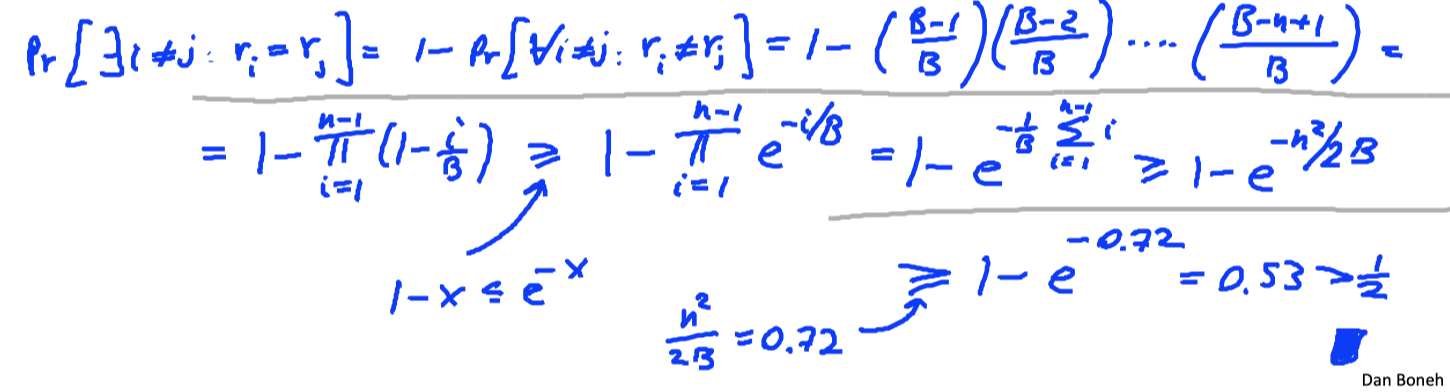### Sample C.R. hash functions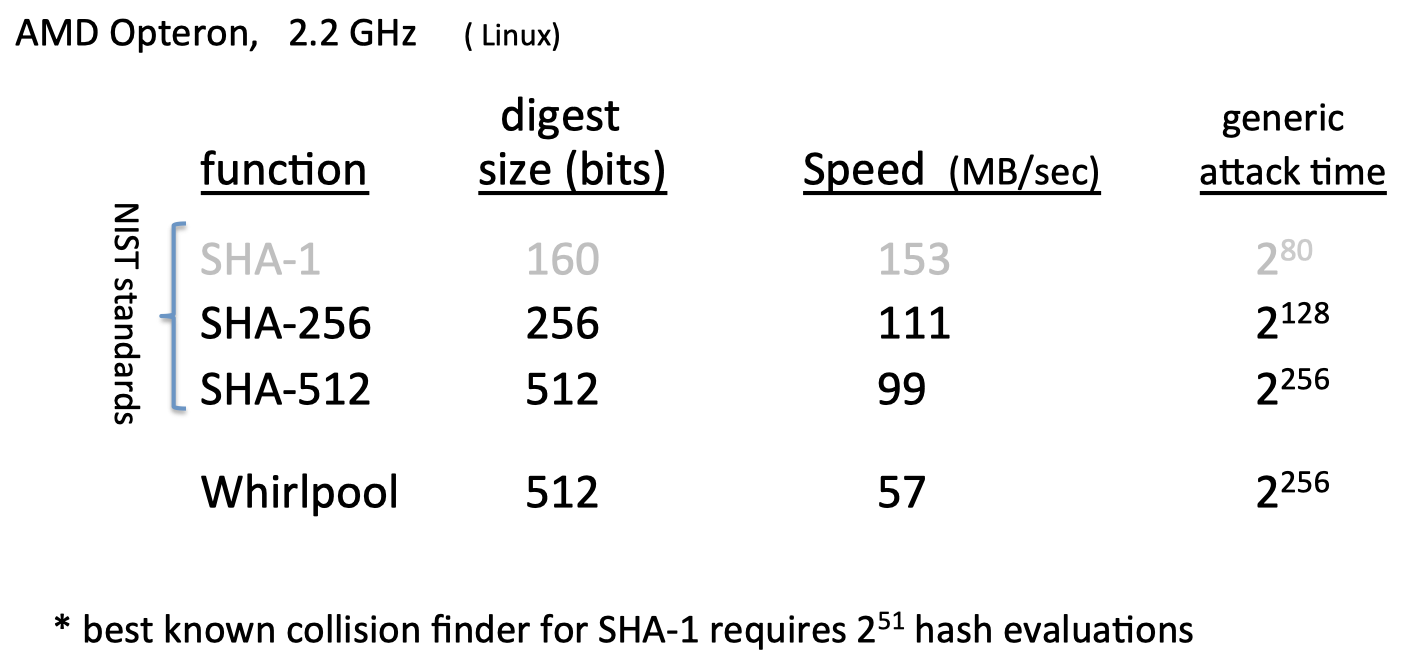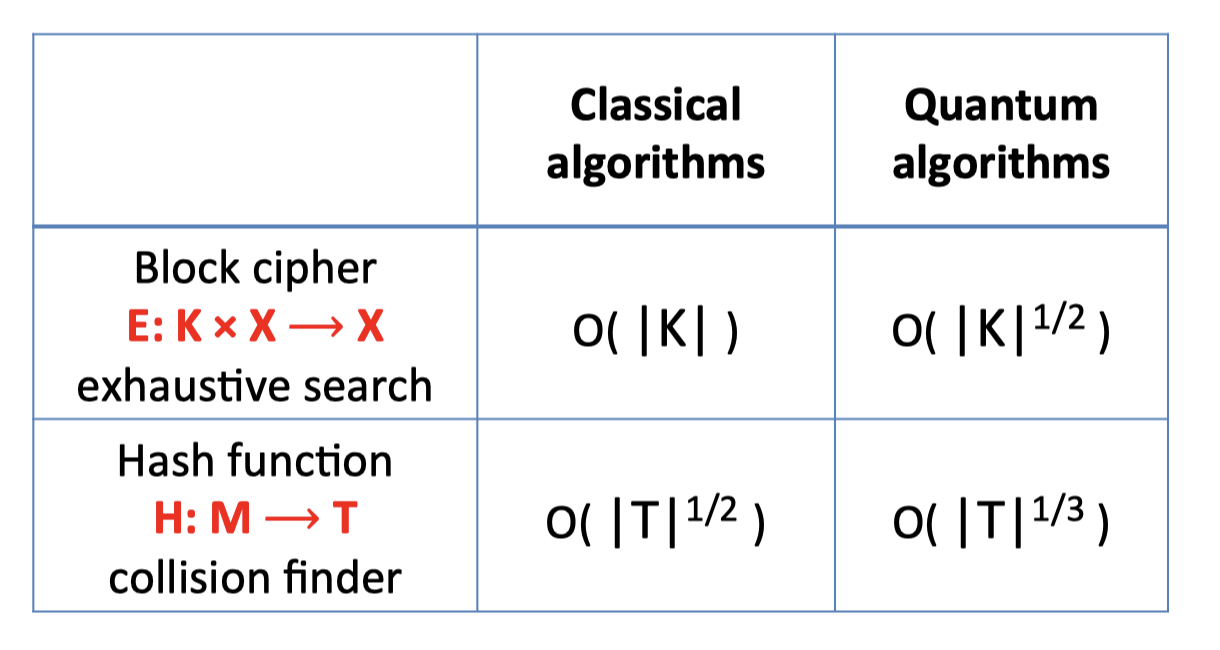### Three way collision

1. n个不同的数字中，有 $n\times(n-1)$ 个pair$(x, y)$ .
2. 而对于任意值$H(x)$，$y$满足 $H(y)=H(x)$ 的概率为 $1/t$ .
3. 因此应该满足： $n^2=t$

1. n个不同的数字中，有 $\frac{n\times(n-1)\times(n-2)}{3!}$ 个pair $(x,y,z)$ .
2. 而对于任意值H(x)，y和z满足 $H(y)=H(z)=H(x)$ 的概率为 $1/t^2$
3. 因此应该满足：$n^3/6 = t^2$

# The Merkle-Damgard Paradigm

## The MD iterated construction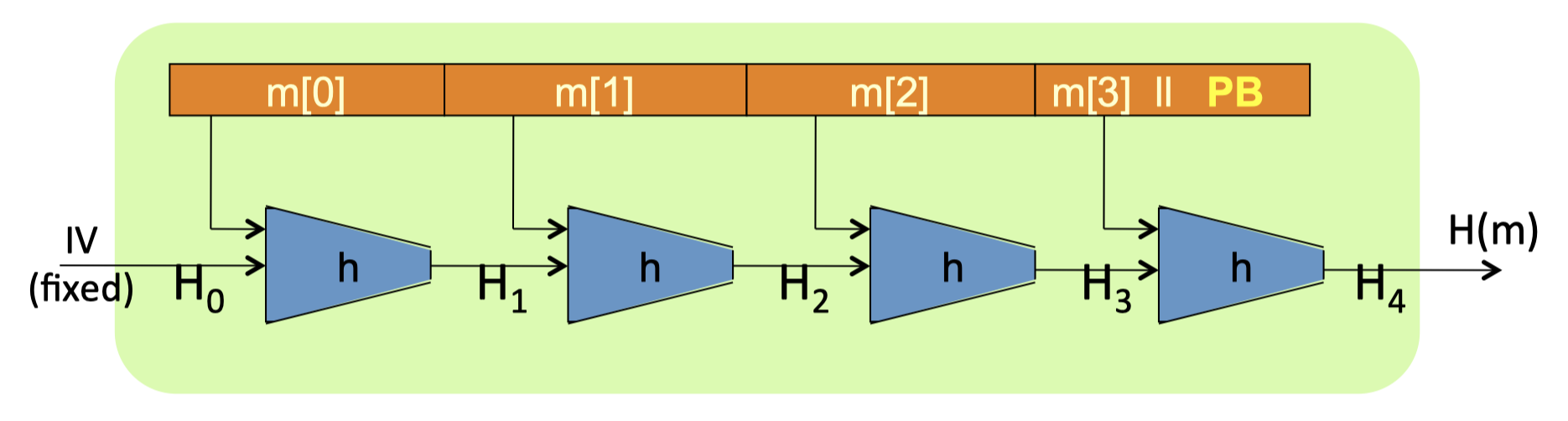$\mathrm{H_i}:$ chaining variables

$\mathrm{PB}:$ padding block （If no space for PB add another block）.## MD collision resistance

Thm: If h is collision resistant then so is H.

【如果压缩函数h是抗哈希的，那么构造出的哈希函数也是抗哈希的】

Proof: collision on H $\Rightarrow$ collision on h

【通过反证法证明，如果能找到H的碰撞，那么也能找到h压缩函数的碰撞】

• 【1】只需要最后一块满足：$\mathrm{h\left(H_{t}, M_{t} | P B\right)=H_{t+1}=H_{r+1}^{\prime}=h\left(H_{r}^{\prime}, M_{r}^{\prime} | P B^{\prime}\right)}$ .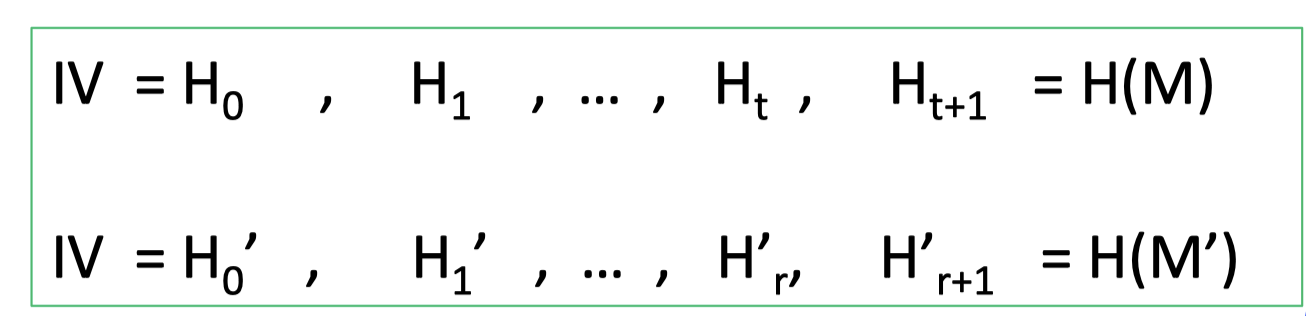• 【1】如果h函数的参数不完全相同，那么就找到了h的碰撞
即 $\mathrm{H_t\ne H_r’}$ or $\mathrm{M_t\ne M_r’}$ or $\mathrm{PB\ne PB’}$ .

• 【2】否则：如果 $\mathrm{H_t= H_r’}$ and $\mathrm{M_t= M_r’}$ and $\mathrm{PB= PB’}$ （可以得出 $t=r$ ）
满足式子：$\mathrm{h\left(H_{t-1}, M_{t-1} \right)=H_{t}=H_{t}^{\prime}=h\left(H_{t-1}^{\prime}, M_{t-1}^{\prime} \right)}$ .

• 【2】同样的，如果h函数的参数不完全相同，就找到了一个h的碰撞：

即 $\mathrm{H_{t-1}\ne H_{t-1}’}$ or $\mathrm{M_{t-1}\ne M_{t-1}’}$ .

• 【*】因此通过迭代的方式，到最后：

• 要么找到h压缩函数的碰撞
• 要么 $\forall i: M_i=M_i’ \Rightarrow M=M’$ ，但这就不满足是H哈希函数的碰撞了。
• 因此，如果能找到H哈希函数的碰撞，就一定能找到h压缩函数的碰撞。

# Constructing Compression Functions

## Comp. func. from a block cipher

block cipher: $\mathrm{E}: \mathrm{K} \times{0,1}^{\mathrm{n}} \longrightarrow{0,1}^{\mathrm{n}}$

Davies-Meyer compression function: $\mathrm{h(H, m)=E(m, H) \oplus H}$

【把消息块m作为密钥】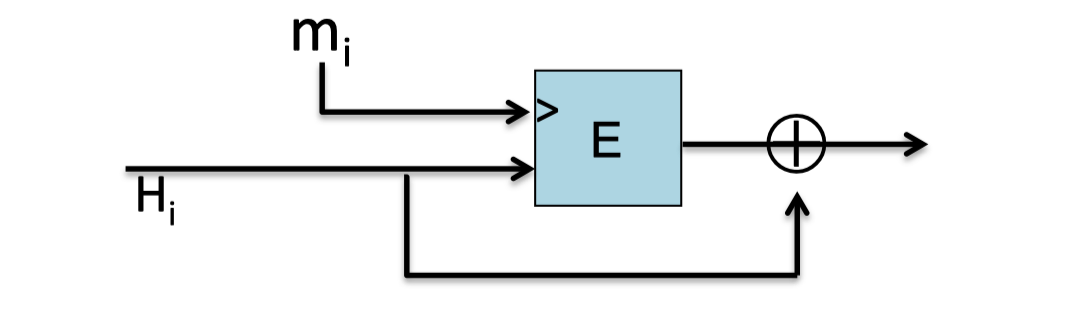Thm: Suppose E is an ideal cipher (collection of |K| random perms.)
Finding a collision $\mathrm{h(H,m)=h(H’,m’)}$ takes $\mathcal{O}(2^{n/2})$ evaluations of (E, D).

【如果E是一个理想的密码，即每一个密钥都对应一个随机置换，那么通过生日攻击，找到一个碰撞需要 $\mathcal{O}(2^{n/2})$ 次(E, D)的计算。】

Miyaguchi-Preneel:

• $\mathrm{h(H, m)=E(m, H) \oplus H \oplus m}$ (Whirlpool)
• $\mathrm{h(H, m)=E(H \oplus m, m) \oplus m}$
• and so on.

## Case study: SHA-256

SHA-256哈希函数的组成要件：

• Merkle-Damgard function
• Davies-Meyer compression function
• Block cipher: SHACAL-2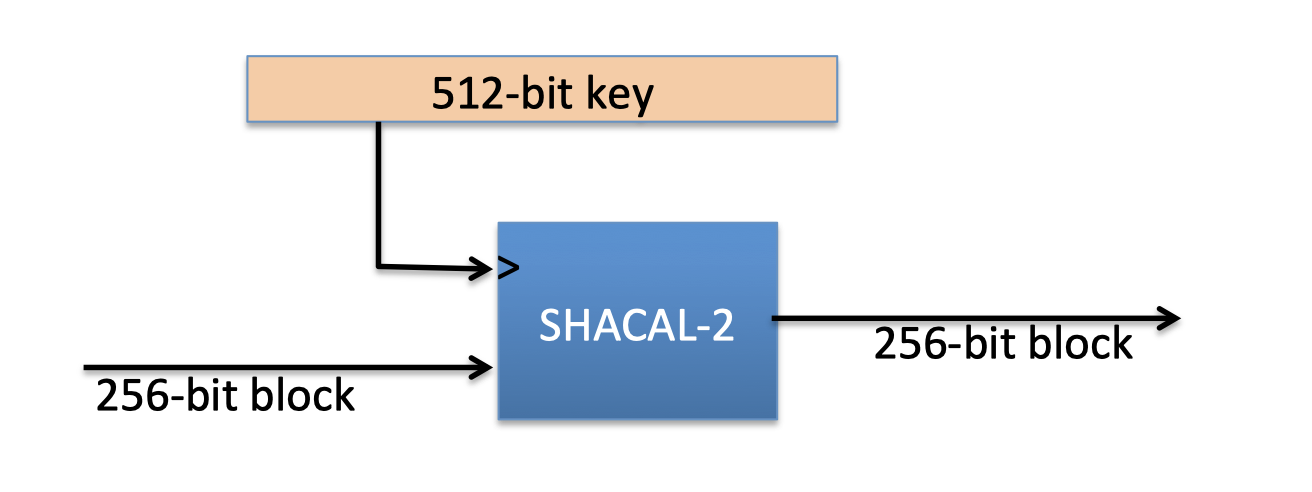## Provable compression functions

Fact: ﬁnding collision for h(.,.) is as hard as solving “discrete‐log” modulo p.

Problem: slow.

# HMAC$\mathrm{\mathrm{H}: X^{\leq L} \longrightarrow T}$ a C.R. Merkle-Damgard Hash Function

Attempt： $\mathrm{s(k, m)=H(k | m)}$

## HMAC: Hash MAC

HMAC可以将任意哈希函数作为黑盒，因此HMAC广泛应用于互联网中的协议。

HMAC:
$$\mathrm{S}(\mathrm{k}, \mathrm{m})=\mathrm{H}(\mathrm{k} \oplus \mathrm{opad} | \mathrm{H}(\mathrm{k} \oplus \mathrm{ipad} | \mathrm{m}))$$

HMAC的结构和基于PRF的NMAC结构很像：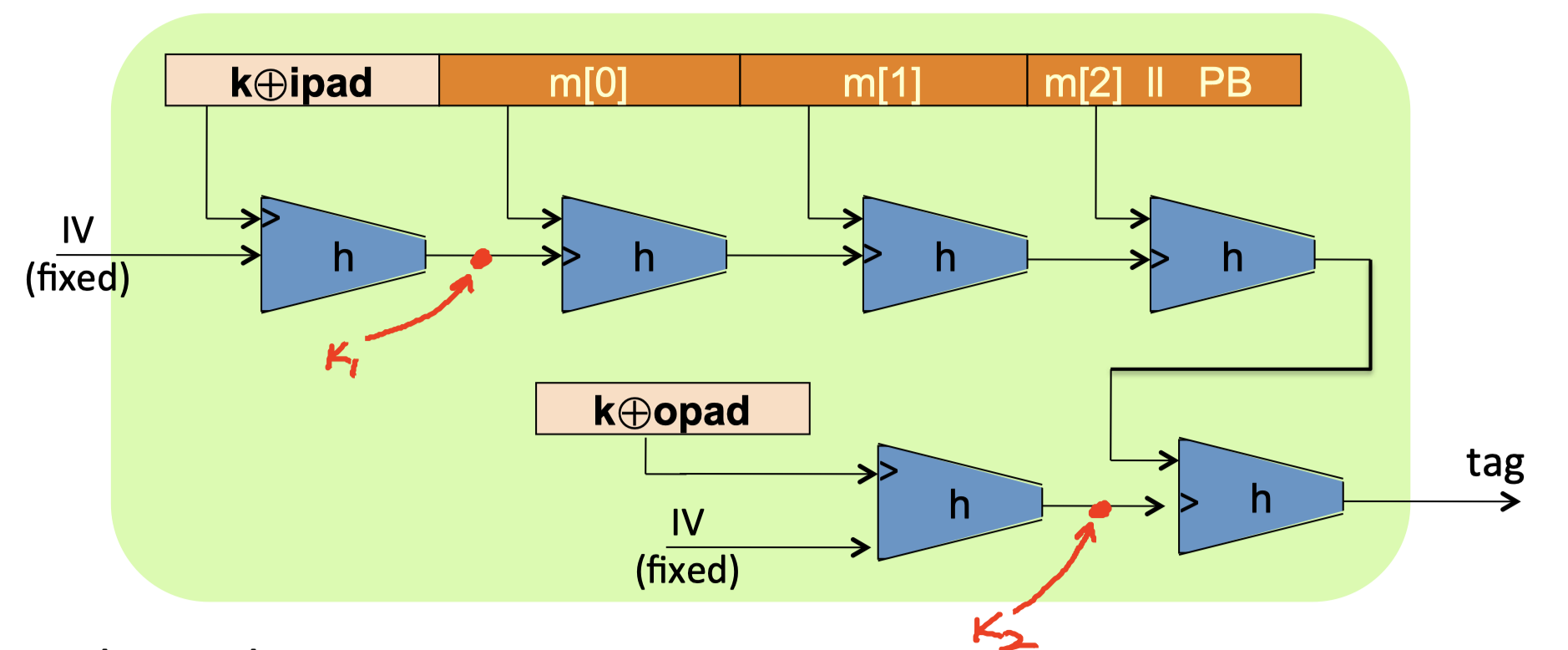• $\mathrm{h(IV,k\oplus ipad)}$ : 是NMAC的 $\mathrm{k_1}$ .
• $\mathrm{h(IV,k\oplus opad)}$ : 是NMAC的 $\mathrm{k_2}$ .
• 主要的不同点在于，HMAC中的两个密钥 $\mathrm{k_1,k_2}$ 是相关的。

# Timing Attacks on MAC VerificationProblem: ‘==’在python中的实现是按字节对比 (byte-by-byte comparison)。

## Timing Attack

Timing attack: 对目标消息m计算tag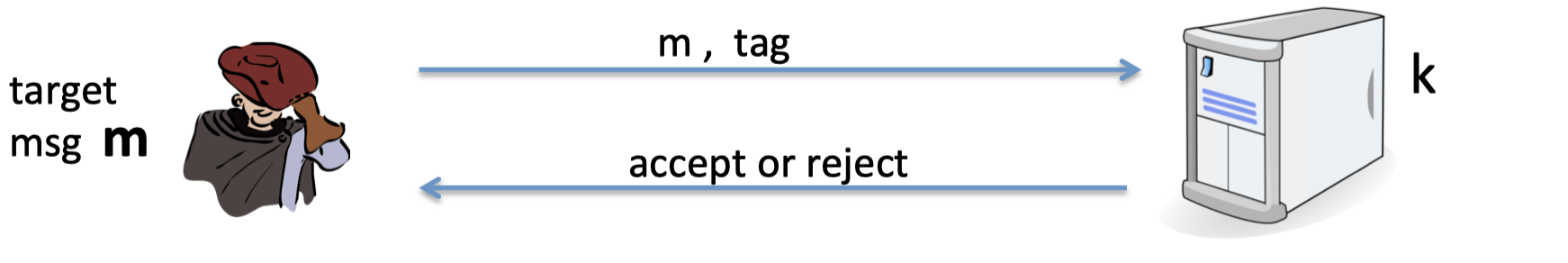1. 向服务器随机请求一个随机tag，记录下验证时间。
2. 遍历随机tag的第一个字节，发送给服务器。当验证时间比第一步中的时间略长时，停止，即找到了第一个字节的值。
3. 重复tag的所有字节，直到找到最终有效的tag。

## Defense 1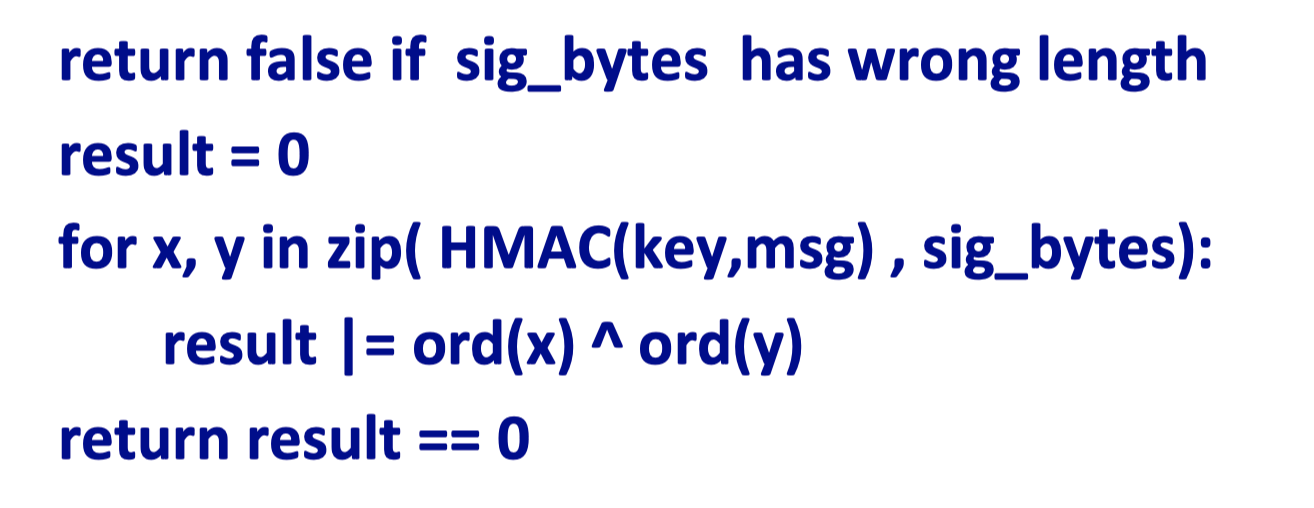## Defense 2Boneh: Don’t implement crypto yourself!

「Cryptography-Boneh」:Collision Resistance

https://f7ed.com/2021/12/28/stanford-integrity2/

f1ed

2021-12-28

2021-12-28